Analog Electronics - 3

# Analog Electronics - 3

Test Description

## 10 Questions MCQ Test GATE ECE (Electronics) 2023 Mock Test Series | Analog Electronics - 3

Analog Electronics - 3 for Electronics and Communication Engineering (ECE) 2023 is part of GATE ECE (Electronics) 2023 Mock Test Series preparation. The Analog Electronics - 3 questions and answers have been prepared according to the Electronics and Communication Engineering (ECE) exam syllabus.The Analog Electronics - 3 MCQs are made for Electronics and Communication Engineering (ECE) 2023 Exam. Find important definitions, questions, notes, meanings, examples, exercises, MCQs and online tests for Analog Electronics - 3 below.
Solutions of Analog Electronics - 3 questions in English are available as part of our GATE ECE (Electronics) 2023 Mock Test Series for Electronics and Communication Engineering (ECE) & Analog Electronics - 3 solutions in Hindi for GATE ECE (Electronics) 2023 Mock Test Series course. Download more important topics, notes, lectures and mock test series for Electronics and Communication Engineering (ECE) Exam by signing up for free. Attempt Analog Electronics - 3 | 10 questions in 30 minutes | Mock test for Electronics and Communication Engineering (ECE) preparation | Free important questions MCQ to study GATE ECE (Electronics) 2023 Mock Test Series for Electronics and Communication Engineering (ECE) Exam | Download free PDF with solutions
 1 Crore+ students have signed up on EduRev. Have you?
Analog Electronics - 3 - Question 1

### When the gate-to-source voltage VGS of a MOSFET with a threshold voltage of 400mV, working in saturation is 900mV, the drain current is observed to be 1mA. Neglecting the channel width modulation effect and assuming that the MOSFET is operating at saturation, the drain current for an applied VGS of 1400mV is

Detailed Solution for Analog Electronics - 3 - Question 1

The MOSFET current is saturation is given by,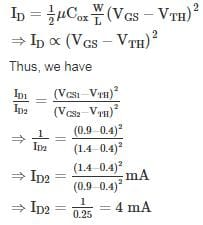*Answer can only contain numeric values
Analog Electronics - 3 - Question 2

### What percentage of current IDSS is the drain current for a JFET, if the gate to source voltage is 65% of the pinch-off voltage.

Detailed Solution for Analog Electronics - 3 - Question 2

VGS = 0.65 Vp

The drain current of JFET is given as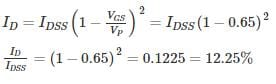Analog Electronics - 3 - Question 3

### The ‘Pinch-off’ voltage of a JFET is 4 V. Then the off gate voltage of JFET is

Detailed Solution for Analog Electronics - 3 - Question 3

The Cut off gate voltage is also known as pinch off voltage at which the drain current becomes zero.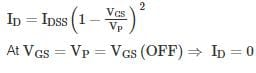*Answer can only contain numeric values
Analog Electronics - 3 - Question 4

A MOSFET operating in saturation mode is shown in the below figure. The drain current is given as ID = ½ (VGS – 0.75)2 mA If VS = 5V, then the value of RS­­­ in kΩ is __________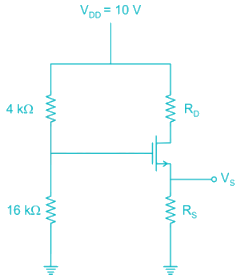Detailed Solution for Analog Electronics - 3 - Question 4

The bias used in the given circuit is voltage divider bias. The gate voltage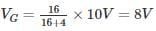∴ Gate source voltage VGS = VG - VS

= 8 – 5

= 3 V

∴ ID = ½ (3 - 0.75)2 mA

≅ 2.53 mA

VS = RSID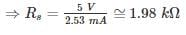*Answer can only contain numeric values
Analog Electronics - 3 - Question 5

For the amplifier shown below, the drain current changes from 5 mA to 7 mA when the gate voltage D changed from -4.0 V to -3.7 V. The voltage gain of the amplifier is _____

Detailed Solution for Analog Electronics - 3 - Question 5

The gain of the amplifier is given by:

Av = - gmrD

gm: trans conductance of transistor

rD: effective AC resistance seen by drain terminal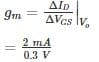gm = 6.66 ms

rD = RD||RL = 6k||6k = 3 kΩ

Av = -6.66 × 10-3 × 3 × 103

Av = -19.98 ≈ 20

Analog Electronics - 3 - Question 6

The NMOS transistors in the circuit below have VT = 1 V, μncox = 120 μA/V2, λ = 0 and L1 = L2 = L3 = 1 μm. The values of width of each of Q1, Q2, Q3 such that the voltage values are as shown in below figure are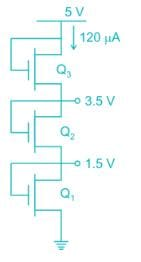Detailed Solution for Analog Electronics - 3 - Question 6

Let ID be the drain current, it will be common for all three transistors. All the transistors are operating in saturation since VD = VG

At transistor Q3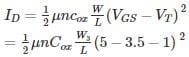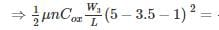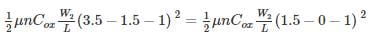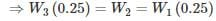Given ID = 120 μA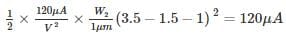⇒ W2 = 2 μm

*Answer can only contain numeric values
Analog Electronics - 3 - Question 7

A common source amplifier biased at ID = 0.25 mA with RD = 20 kΩ and early voltage VA = 50 V and overdrive voltage (VGS – VT)DC = 0.25 V. If the input is fed through 100 kΩ and output are taken from 20 kΩ loads then the overall gain of the amplifier is _____ V/V.

Detailed Solution for Analog Electronics - 3 - Question 7

The Ac equivalent circuit can be drawn as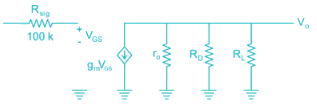ID = 0.25 mA; VA = 50 V; Vov (= VGs - VT) = 0.25 V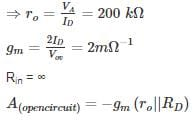(∵ rO >> RD)

= -36.36 V/V

Av(Overall gain) = -gm(R|| r|| RL)

= -2 mΩ-1 × 9.53 kΩ

≃ -19 V/V

Analog Electronics - 3 - Question 8

The transistor I the circuit is shown below has parameters IDSS = 8 mA and VP = -4 V. The value of VDS is___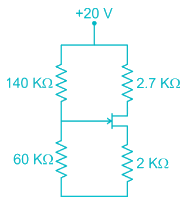Detailed Solution for Analog Electronics - 3 - Question 8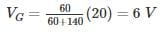Assume the transistor in saturation,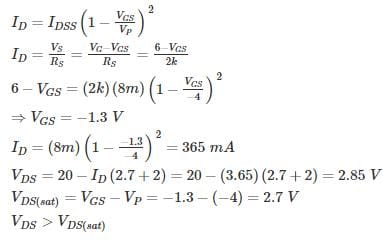Assumption is correct.

Hence VDS = 2.85 V.

*Answer can only contain numeric values
Analog Electronics - 3 - Question 9

The small signal resistance (dVC/dID) of the n-channel MOSFET shown in figure below at bias voltage VC = 2V is (in kΩ) _________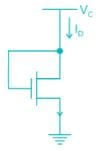Given μnCox W/L = 40μ A/V2

VT = 1 V

Detailed Solution for Analog Electronics - 3 - Question 9

Since VDS = VGS the device is in saturation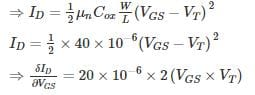= 40 × 10-6 (2 - 1)

Hence small signal resistance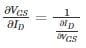= 25 kΩ

*Answer can only contain numeric values
Analog Electronics - 3 - Question 10

In the circuit shown below the parameters are gm =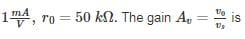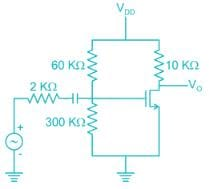Detailed Solution for Analog Electronics - 3 - Question 10

The small signal equivalent circuit is as shown below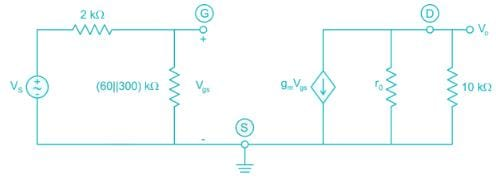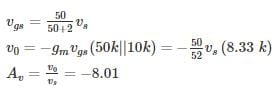## GATE ECE (Electronics) 2023 Mock Test Series

20 docs|263 tests
 Use Code STAYHOME200 and get INR 200 additional OFF Use Coupon Code
Information about Analog Electronics - 3 Page
In this test you can find the Exam questions for Analog Electronics - 3 solved & explained in the simplest way possible. Besides giving Questions and answers for Analog Electronics - 3, EduRev gives you an ample number of Online tests for practice

## GATE ECE (Electronics) 2023 Mock Test Series

20 docs|263 tests